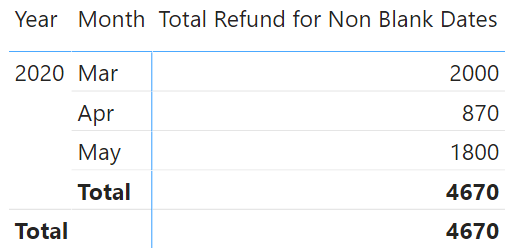Often you’d find yourself in the need to calculate a measure, where values in certain column are non blank. The trick that I am about to share was actually a serendipitous discovery whilst I was on to something else in DAX.

I am sure if you haven’t been purposely using it, you’d find it pretty cool too!

### Consider this simple Data Model

Although the Sales Data (below) is a bit broken with missing values but that is intentional.And it is related with the Calendar table with standard columns.Now consider the following Measure

`Total Sales = SUM(Sales[Value])`

Seeing this across Years and Months will show up like thisSince our Sales data had blank dates it would obviously show unallocated blank values.

But what if I want to – Calculate Sales for Non Blank Dates Only?

### Calculate for Non Blank Dates

If I revise my measure to this

```Total Sales for Non Blank Dates =
CALCULATE(
[Total Sales],
Sales[Sales Date]
)```

and then see it across Years and Month and voila the blank values are gone!Notice the subtlety

1. In the second argument of the CALCULATE function above, I haven’t written any sort of condition such as Sales[Sales Date] <> BLANK().
2. All I have done is write the Sales[Sales Date] column and CALCULATE function will magically calculate sales for non blank values the Sales Date column.
3. Brilliance! 😎

After this serendipity I went on to explore a few more things about this CALCULATE Function feature.

### Calculate for Non Blank Values

The refunded initiated column in our data shows 1 in case of a refund or a blank otherwise. I then wrote the following measure to calculate sales which were refunded for non blank dates.

```Total Refund for Non Blank Dates =
CALCULATE(
[Total Sales],
Sales[Sales Date],
Sales[Refund Initiated]
)```

results..• It works the same, intelligently filtering out the dates and refunded initiated for non blank values.
• Notice that, I still haven’t written any condition in the 2nd and 3rd argument of CALCULATE.
• All I have done is just to mention the column names and it works!

Next up I thought to test this with Boolean – True / False Values

### Calculate Non Blank Values for Boolean

I created a column in the Calendar Table to check if the current date is a weekend or not. If you are interested, here is the DAX formula that returned either a true or false (boolean).

```Weekend Column =
WEEKDAY('Calendar'[Date],2) > 5```

I then wrote another measure to calculate sales for the weekends only.

```Sales for Weekend =
CALCULATE(
SUM(Sales[Value]),
'Calendar'[Weekend Column]
)```

Again, it works calculating the values for the weekend.My last test was to find, if this works for text values or not.

### Calculate Non Blank Values for Text

Consider the Add-on column the sales table which displays a “yes”, if the customer purchased an add-on else blank. So I wrote another measure to calculate Sales for Add-ons for Non Blank dates

```Total Sales Addons for Non Blank Dates =
CALCULATE(
[Total Sales],
Sales[Sales Date],
)```
• Just like the other times, I use the same pattern of mentioning just the column names in the Calculate function… and it doesn’t work. Why??
• Concluding with the fact that this trick works with non blank dates, non blank numbers even boolean (true or false) but not non blank text values.

To get over the problem I came up with a simple workaround of adding ISTEXT around the Add-Ons column. Which actually converts it into a boolean and voila again!

```Total Sales Addons for Non Blank Dates =
CALCULATE(
[Total Sales],
Sales[Sales Date],
)```

This fella works just fine 🙂 See this### More DAX Awesomeness !

1. Slab or Tier based Calculations in DAX
2. Calculating Fiscal Weeks in Power BI – Week Counting that starts from April
3. Sort by Column – Interesting Examples
4. Calculate Same Period Last Year only up-to a certain Date
5. Performing Top Product Analytics
6. Performing Bottom Product Analytics

Tagged In .